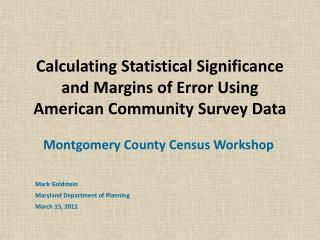DownloadDownload PresentationCalculating Statistical Significance and Margins of Error Using American Community Survey Data

# Calculating Statistical Significance and Margins of Error Using American Community Survey Data

Télécharger la présentation## Calculating Statistical Significance and Margins of Error Using American Community Survey Data

- - - - - - - - - - - - - - - - - - - - - - - - - - - E N D - - - - - - - - - - - - - - - - - - - - - - - - - - -
##### Presentation Transcript

1. Calculating Statistical Significance and Margins of Error Using American Community Survey Data Montgomery County Census Workshop Mark Goldstein Maryland Department of Planning March 15, 2011

2. OR … Making it Easy…to Use ACS Data!

3. ACS Sample Size for MarylandHousing Units Three-year sample size (2007-2009) = 4.1% Five-year sample size = 6.9% Decennial Census Long Form = 16.7%

4. Sampling Error & Standard Error • Sampling error occurs when estimates are derived from a sample rather than a census (complete count) of the population. • Standard error is an estimate of sampling error – how precise the survey estimates are to the true population you are trying to measure

5. Sampling Error & Margin of Error • Margin of Error = standard error for a given confidence interval (typically 90 percent). A measure of the precision of the estimate at a given confidence interval • Sampling error in the ACS is reported as the estimate “plus or minus” the margin of error

6. Margin of Error (MOE) • MOE = 1.645 * Standard error where 1.645 is used for the 90 pct confidence interval (if want 95%, use 1.960; for 99% use 2.576) • Use the MOE to construct the Lower and Upper bounds around the estimate • Lower Bound = (estimate – MOE) • Upper Bound = (estimate + MOE)

7. Median Household Income Estimate for Montgomery Co from the 2009 ACS 90 % C.I. = \$94,420 +/- \$2,347 = \$92,073 to \$96,767

8. 90 Percent Confidence Interval • There is a nine-out-of ten, or 90 % chance, that the interval contains the “true” value that you would have gotten from a full census

9. Why you should care about Margins of Error • Lets you know how good the data is • Saves you from drawing erroneous conclusions. • Helps you decide how confident you can be about the assertions you make

10. First Example: Comparing Two Estimates • If have two estimates, need to determine if the apparent differences are “real” (i.e. are statistically significant) • Quick and dirty method is to “eye ball” whether the confidence intervals of the two estimates overlap

11. Comparing Two Estimates(the easy way) • If the confidence intervals of two estimates do not overlap, then the difference between the two estimates are statistically significant • If the confidence intervals of two estimates do overlap, then the difference between the two estimates may or may not be statistically significant (will need to test)

12. 2009 Median Household Income Estimates Source: 2009 American Community Survey

13. Comparing Two Estimates • Need to do a formal test of statistical significance if the confidence intervals do overlap

14. Testing Statistical Significance • Absolute value of Difference = ABS(X –Y) • SE(x) = MOEx/1.645 • SE(y) = MOEy/1.645 • SE(x-y) = • MOE (x-y) = SE (x-y)*1.645 • ABS(X-Y) <> MOE (x-y)

15. Testing Statistical Significance • If ABS(X-Y) > MOE(x-y), then the difference between the two estimates are statistically significant • If ABS(X-Y) < MOE (x-y), then the difference between the two estimates are NOT statistically significant

16. Go to Statistical Calculations Excel File! (significance test worksheet) Thank you Dr. Lenny Gaines, New York State Data Center

17. Testing a Difference Over Time

18. Go to Statistical Calculations Excel File! (significance test worksheet)

19. Second Example – Calculate MOE of the sum of two or more estimates Top Six Places for Foreign Born in Montgomery County (2005-2009)

20. Second Example – Margin of Error of a Sum • Calculate the standard error of the sum: • SE (x1+x2+x3)= 2. Calculate the Margin of error of the sum: MOE(x1+x2+x3) = SE(x1+x2+x3)*1.645

21. Second Example – Calculate MOE of the sum of two or more estimates Top Six Places for Foreign Born in Montgomery County (2005-2009)

22. Third Example – Margin of Error of a Proportion(e.g., What proportion of Montgomery’s foreign born population are in the top six places?) X = number of foreign born in top six places Y = number of foreign born in Montgomery County i.e. X is a subset of Y OR MOE (x/y) = SE(x/y)*1.645

23. Go to Statistical Calculations Excel File! (StdErr of a proportion worksheet)

24. Fourth Example – Margin of Error of a Ratio(e.g. What is the ratio of median income of foreign born to native born households in Montgomery Co. X = median income of foreign born households Y = median income of native born households i.e., X is NOT a subset of Y MOE (x/y) = SE(x/y)*1.645

25. Go to Statistical Calculations Excel File! (Std Err of a ratio worksheet)

26. Thank You…. Hope we made it (somewhat) easier